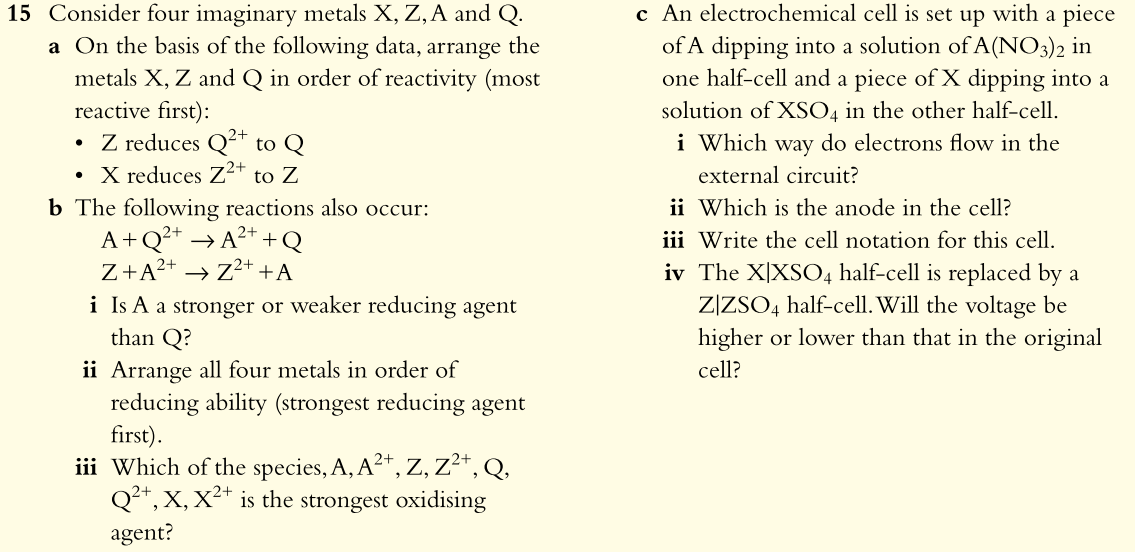# Question Solved1 Answer15 Consider four imaginary metals X, Z, A and Q. a On the basis of the following data, arrange the metals X, Z and Q in order of reactivity (most reactive first): • Z reduces Q2+ to Q • X reduces z2+ to Z b The following reactions also occur: A+Q2+ → A2+ +Q Z+A2+ → Z2+ +A i Is A a stronger or weaker reducing agent than Q? ii Arrange all four metals in order of reducing ability (strongest reducing agent first) iii Which of the species, A, A2+, Z, Z2+, Q, Q2+, X, X2+ is the strongest oxidising agent? c An electrochemical cell is set up with a piece of A dipping into a solution of A(NO3)2 in one half-cell and a piece of X dipping into a solution of XSO4 in the other half-cell. i Which way do electrons flow in the external circuit? ii Which is the anode in the cell? jii Write the cell notation for this cell. iv The X|XSO4 half-cell is replaced by a ZIZSO4 half-cell. Will the voltage be higher or lower than that in the original cell?Transcribed Image Text: 15 Consider four imaginary metals X, Z, A and Q. a On the basis of the following data, arrange the metals X, Z and Q in order of reactivity (most reactive first): • Z reduces Q2+ to Q • X reduces z2+ to Z b The following reactions also occur: A+Q2+ → A2+ +Q Z+A2+ → Z2+ +A i Is A a stronger or weaker reducing agent than Q? ii Arrange all four metals in order of reducing ability (strongest reducing agent first) iii Which of the species, A, A2+, Z, Z2+, Q, Q2+, X, X2+ is the strongest oxidising agent? c An electrochemical cell is set up with a piece of A dipping into a solution of A(NO3)2 in one half-cell and a piece of X dipping into a solution of XSO4 in the other half-cell. i Which way do electrons flow in the external circuit? ii Which is the anode in the cell? jii Write the cell notation for this cell. iv The X|XSO4 half-cell is replaced by a ZIZSO4 half-cell. Will the voltage be higher or lower than that in the original cell?
More
Transcribed Image Text: 15 Consider four imaginary metals X, Z, A and Q. a On the basis of the following data, arrange the metals X, Z and Q in order of reactivity (most reactive first): • Z reduces Q2+ to Q • X reduces z2+ to Z b The following reactions also occur: A+Q2+ → A2+ +Q Z+A2+ → Z2+ +A i Is A a stronger or weaker reducing agent than Q? ii Arrange all four metals in order of reducing ability (strongest reducing agent first) iii Which of the species, A, A2+, Z, Z2+, Q, Q2+, X, X2+ is the strongest oxidising agent? c An electrochemical cell is set up with a piece of A dipping into a solution of A(NO3)2 in one half-cell and a piece of X dipping into a solution of XSO4 in the other half-cell. i Which way do electrons flow in the external circuit? ii Which is the anode in the cell? jii Write the cell notation for this cell. iv The X|XSO4 half-cell is replaced by a ZIZSO4 half-cell. Will the voltage be higher or lower than that in the original cell?